• RSA密码算法
千次阅读
2021-06-11 15:41:37

# 1. RSA非对称加密原理

网上一大把，这里推荐一篇比较好的博客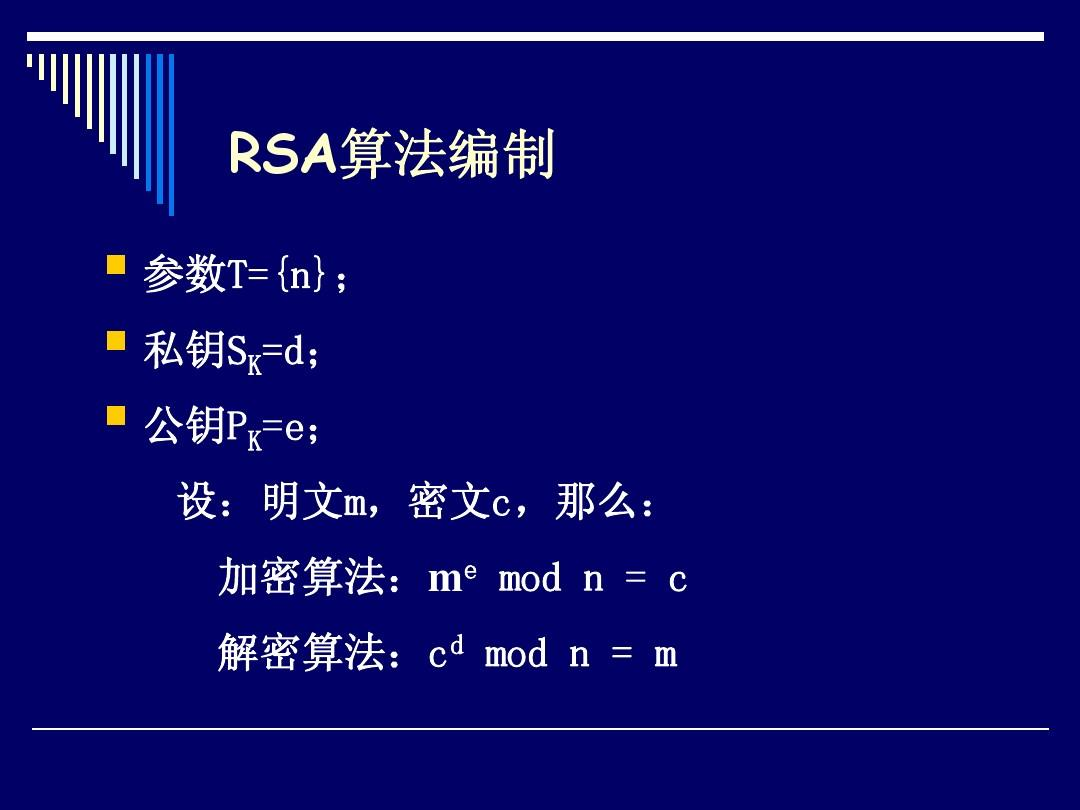# 2. C++ 随机生成密钥版本

#include <iostream>
#include <stdio.h>
#include <stdlib.h>
#include <ctype.h>
#include<string.h>
#include <math.h>
#include<algorithm>

using namespace std;
typedef long long ll;

// e是公钥
// d是私钥

ll e, d, n;

ll gcd(ll a, ll b)  //求最大公约数
{
ll c = 0;
if(a<b) swap(a,b);
c = b;
do
{
b = c;
c = a%b;
a = b;
}
while (c != 0);
return b;
}

// 0不是 1是
ll isPrime(ll i) //判断i是否是素数
{
ll flag=0;
for(ll a=2; a<i; a++)
{
if(i%a==0)
{
flag=1;
break;
}
}
if(flag==1) return 0;
else return 1;

}

ll myPow(ll a, ll b, ll n)  //求a^b mod n
{
ll y;

/*使用二进制平方乘法计算 pow(a,b) % n*/
y=1;

while(b != 0)
{
/*对于b中的每个1，累加y*/

if(b & 1)
y = (y*a) % n;

/*对于b中的每一位，计算a的平方*/
a = (a*a) % n;

/*准备b中的下一位*/
b = b>>1;
}

return y;

}

void extgcd(ll a,ll b,ll& d,ll& x,ll& y)
{
if(!b)
{
d=a;
x=1;
y=0;
}
else
{
extgcd(b,a%b,d,y,x);
y-=x*(a/b);
}
}

ll ModularInverse(ll a,ll b)  //获取(1/a)mod b的结果
{
ll d,x,y;
extgcd(a,b,d,x,y);
return d==1?(x+b)%b:-1;

}

void KeyGeneration()  //获取公钥密钥
{
ll p, q;
ll phi_n;

do
{
do
p = rand();
while (p % 2 == 0);

}
while (!isPrime(p)); 	// 得到素数 p

do
{
do
q = rand();
while (q % 2 == 0);
}
while (!isPrime(q)); 	// 得到素数q

n = p * q;
phi_n = (p - 1) * (q - 1);

do
e = rand() % (phi_n - 2) + 2; // 1 < e < phi_n
while (gcd(e, phi_n) != 1);

d = ModularInverse(e,phi_n);
}

// 一位一位地输出加密的结果
ll Encryption(ll value)  //加密
{
ll cipher;
cipher = myPow(value, e, n);
cout<<cipher;
return cipher;
}

// 一位一位地输出解 密的结果
void Decryption(ll value)  //解密
{
ll decipher;
decipher = myPow(value, d, n);
cout<<decipher;
}
int main()
{
/******

对6位的数字进行稳定加密

******/
ll num;
cout<<"请输入要加密的明文数字,ctrl+c结束"<<endl;
while(cin>>num){
ll de;
cout<<"输入的明文为"<<num<<endl;
KeyGeneration();  //获取公钥密钥
cout<<"加密密钥："<<e<<endl;
cout<<"加密结果为:";
de = Encryption( num );
cout<<"\n私钥为："<<d;
//		cout<<"de="<<de<<endl;
cout<<"\n解密结果";
Decryption(de);
cout<<"\n-------------"<<endl;
}
return 0;
}


# 3. 密钥固定e=13版本

#include <iostream>
#include <stdio.h>
#include <stdlib.h>
#include <ctype.h>
#include<string.h>
#include <math.h>
#include<algorithm>
using namespace std;
typedef long long ll;

char map = {
'a', 'b', 'c', 'd', 'e','f','g',
'h', 'i', 'j', 'k', 'l', 'm', 'n',
'o', 'p', 'q', 'r', 's', 't',
'u', 'v', 'w', 'x', 'y', 'z'
};
//cp存密文， ep存明文
char cp, ep;
int lcp , lep ;

ll getNum(char a, char b){
int aa,bb;
for(int i=0; i<30; i++){
if(map[i]==a){
aa = i;
}
if(map[i]==b){
bb = i;
}
}
return aa*100+bb;
}
// flag = 1, 解密转换得到的是明文
// flag = 2 加密转换得到的是密文
void toChar(ll num, int flag = 1){
int a = num/100;
int b = num%100;
a %= 26;
b %= 26;
cout<<map[a]<<map[b]<<" "<<endl;
if(flag==1){
ep[lep++] = map[a];
ep[lep++] = map[b];
}
else if(flag==2){
cp[lcp++] = map[a];
cp[lcp++] = map[b];
}
}

// e是公钥
// d是私钥

ll e, d, n;

ll gcd(ll a, ll b)  //求最大公约数
{
ll c = 0;
if(a<b) swap(a,b);
c = b;
do
{
b = c;
c = a%b;
a = b;
}
while (c != 0);
return b;
}

// 0不是 1是
ll isPrime(ll i) //判断i是否是素数
{
ll flag=0;
for(ll a=2; a<i; a++)
{
if(i%a==0)
{
flag=1;
break;
}
}
if(flag==1) return 0;
else return 1;

}

ll myPow(ll a, ll b, ll n)  //求a^b mod n
{
ll y;

/*使用二进制平方乘法计算 pow(a,b) % n*/
y=1;

while(b != 0)
{
/*对于b中的每个1，累加y*/

if(b & 1)
y = (y*a) % n;

/*对于b中的每一位，计算a的平方*/
a = (a*a) % n;

/*准备b中的下一位*/
b = b>>1;
}

return y;

}

void extgcd(ll a,ll b,ll& d,ll& x,ll& y)
{
if(!b)
{
d=a;
x=1;
y=0;
}
else
{
extgcd(b,a%b,d,y,x);
y-=x*(a/b);
}
}

ll ModularInverse(ll a,ll b)  //获取(1/a)mod b的结果
{
ll d,x,y;
extgcd(a,b,d,x,y);
return d==1?(x+b)%b:-1;

}

void KeyGeneration()  //获取公钥密钥
{
ll p, q;
ll phi_n;

p = 43; q = 59, e=13;
n = p * q;
phi_n = (p - 1) * (q - 1);

d = ModularInverse(e,phi_n);
}

// 一位一位地输出加密的结果
ll Encryption(ll value)  //加密
{
ll cipher;
cipher = myPow(value, e, n);
cout<<"加密得到的数字="<<cipher<<"\t加密得到的字母=";
toChar(cipher, 2);
return cipher;
}

// 一位一位地输出解 密的结果
void Decryption(ll value)  //解密
{
ll decipher;
decipher = myPow(value, d, n);
cout<<"解密得到的数字="<<decipher<<"\t解密得到的字母=";
toChar(decipher, 1);
}
int main()
{
lcp = 0, lep = 0;
char st[] = "cybergreatwall";
KeyGeneration();
cout<<"e="<<e<<" d="<<d<<" n="<<n <<endl;
int len = strlen(st);
//	cout<<st<<" "<<len<<endl;
for(int i=0; i<len; i+=2){
cout<<"加密的明文="<<st[i]<<st[i+1]<<endl;
ll num = getNum(st[i], st[i+1]);
cout<<"明文对应的数字"<<num<<endl;
ll de = Encryption( num );
Decryption(de);
}
cp[lcp] = '\0';
ep[lep] = '\0';
cout<<"加密的结果为"<<cp<<endl;
cout<<"解密的结果为:"<<ep<<endl;

return 0;
}



完结撒花，cv可用
更多相关内容
• ## RSA密码算法

千次阅读 2019-10-31 08:56:52
介绍：本篇文章主要介绍RSA密码算法的流程、基本定理及其实现难点说明。 一.RSA密码算法 1.安全基础 RSA公钥密码体制的理论基础是数论中的大整数因子分解的困难性，即求两个大素数的乘积，在计算机上很容易实现，...

介绍：本篇文章主要介绍RSA密码算法的流程、基本定理及其实现难点说明。

## 一.RSA密码算法

#### 1.安全基础

RSA公钥密码体制的理论基础是数论中的大整数因子分解的困难性，即求两个大素数的乘积，在计算机上很容易实现，但是，要将一个大整数分解成两个大素数的乘积，在计算机上很难实现。

#### 2.算法流程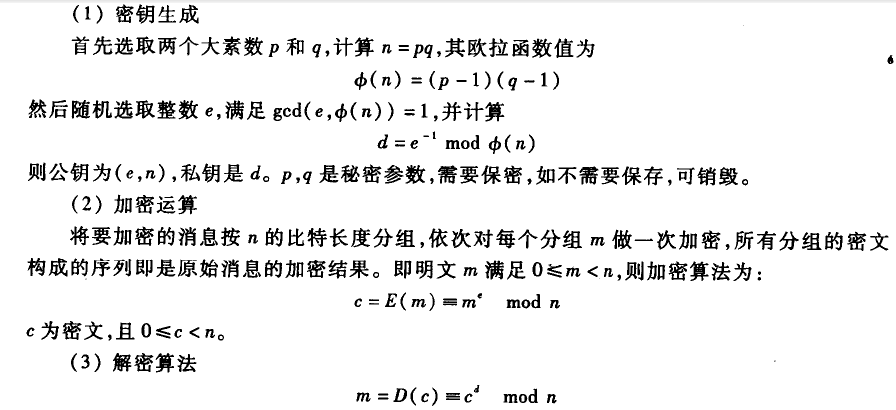注：由于现代计算机计算性能的提高，要求n的比特长度不低于512，现在使用的RSA算法中一般使用的长度一般为512/1024/2048.每次加密过程中要求明文m小于n。

#### 3.算法证明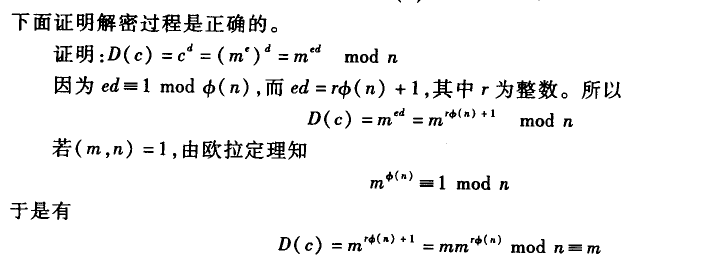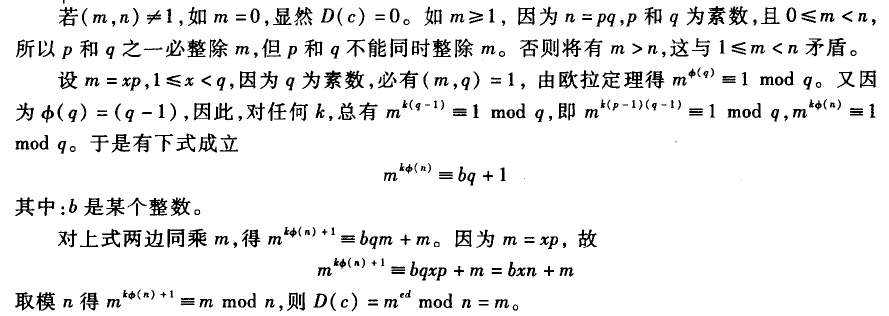#### 4.欧拉定理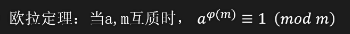#### 5.RSA实例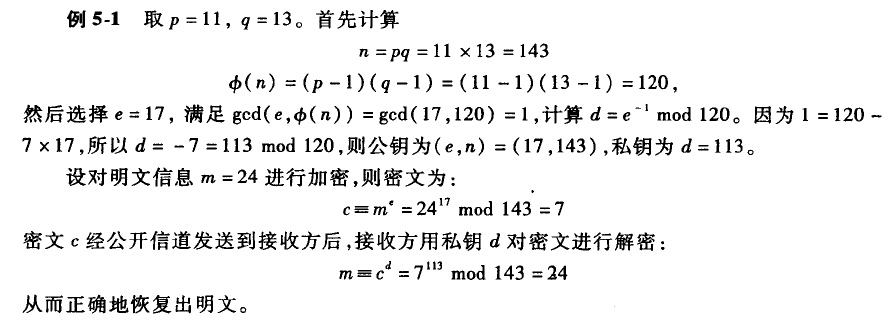## 二.重难点说明

#### 1.大素数选择

在RSA算法中，p、q是两个大素数，这样就涉及到选取大素数的问题。特别的，例如在1024位的RSA算法中，我们可以设计这两个大素数分别为512位（因为两个512bit长度数的乘积为2013或1024bit，而我们需要的是1024bit，故在设计p和q时可将最高的几位固定为1，这样乘积就为1024bit了）。可以通过下面的定理证明一个整数是否为素数，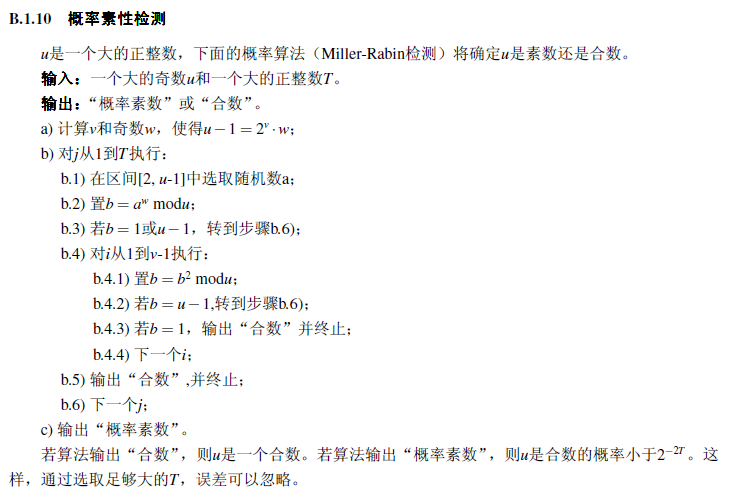#### 2.公私钥产生

1）公钥产生
我们生成公钥e时，要求e与n的欧拉函数φ（n）互素，我们可以通过欧几里得算法验证互素与否。也可以通过构造一个一个素数e，因为e与非倍点的任何数都是互素的，故是符合要求的。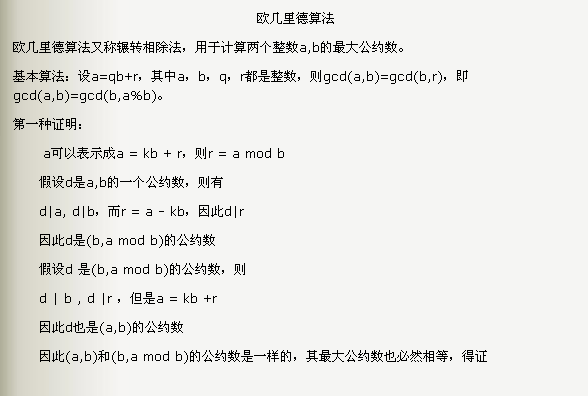while(b!=0)
{
r = a % b;
a = b;
b = r;
}
//最终的a即为原a,b最大公约数


2）私钥产生
私钥d符合要求d = e^(-1)modφ(n)，求逆可以通过拓展欧几里得算法求得。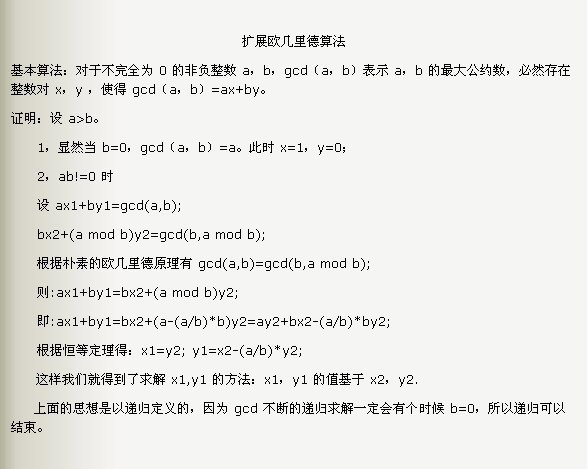int exGcd(int a,int b,int &x,int &y)
{
if(b==0)
{
x = 1;
y = 0;
return a;
}
int r = exGcd(b,a%b,x,y);
t = x; x = y;
y = t-a/b*y;
return r;
}


#### 3.加解密

1）加密
在加密过程中，加密者是不知道n的分解因子p、q，故无法使用中国剩余定理，只能通过常规的模幂运算。
2）解密
方法1：和加密流程一样，使用常规的模幂运算求解。
方法2：基于中国剩余定理（CRT）求解

m = c^d mod n，n = pq，这样就可以通过中国剩余定理将其变换为求解m，使得m = c^d mod p,且m = c^d mod q，这样就可以通过中国剩余 定理来求解。

中国剩余定理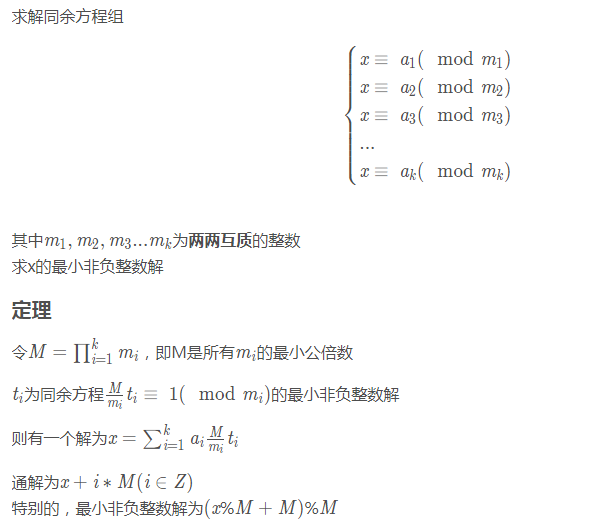通俗写法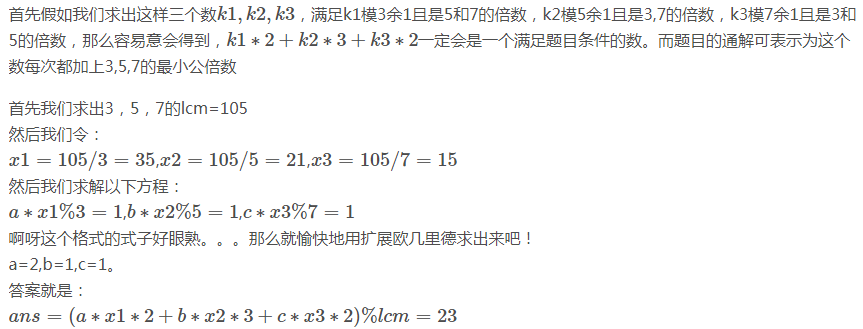拓展：拓展中国剩余定理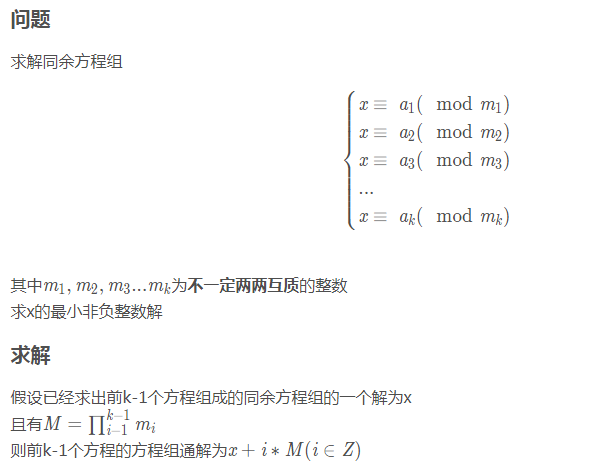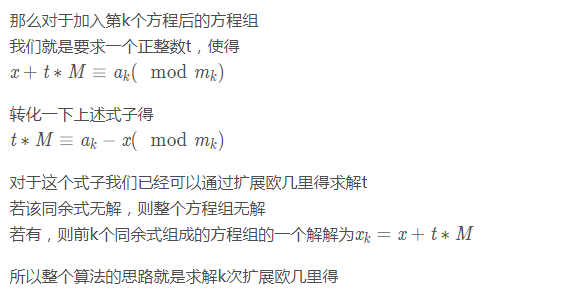注：本文档部分参考自别的博客，侵删。

展开全文公钥加密
• 大数据-算法-RSA密码算法的格攻击技术研究.pdf
• RSA密码算法的功耗轨迹分析及其防御措施.doc
• DES_3DES_AES_IDES_RSA密码算法比较手册
• C/C++语言实现的RSA算法，流程+代码，附有完整的文档
• 基于Python的RSA密码算法的设计与实现.pdf
• 介绍了用于快速计算高次多项式值的“秦九韶算法”，并用类似思路分析了RSA算法中方幂模快速实现算法，最后给出了该算法的具体实现。算法分析和实验结果证明，该算法的计算量不会随着指数的快速增大而增大，通过精心...
• RSA算法能同时用于加密和数字签名，并能实现密钥分发功能，也易于理解和操作.它的安全性依赖于大数分解，但是否等同大数分解网络安全
• 现代密码RSA实现的C语言代码，仅供参考，不足之处也请指教。
• RSA密码算法检测，支持密钥生成、加解密，支持密钥三元组，五元组
• 1） 学习RSA密码算法的原理 2） 学习RSA密码算法的编程实现 【实验原理】 RSA公钥加密算法是1977年由Ron Rivest、Adi Shamirh和LenAdleman在美国麻省理工学院开发的。RSA算法基于一个十分简单的数论事实：将两个...

【实验目的】

1） 学习RSA密码算法的原理

2） 学习RSA密码算法的编程实现

【实验原理】

算法原理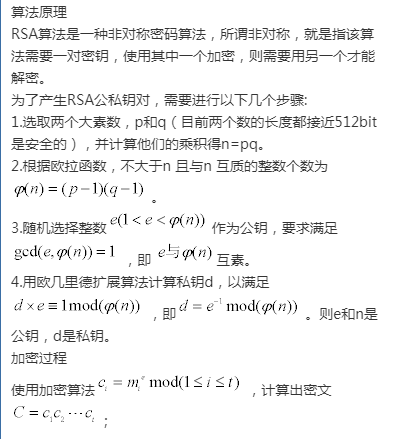解密过程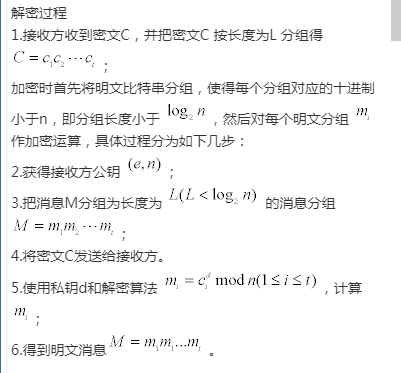算法参数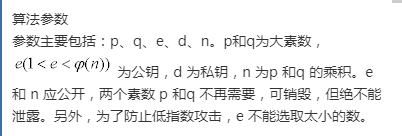算法流程

（1）RSA密钥生成部分代码流程图：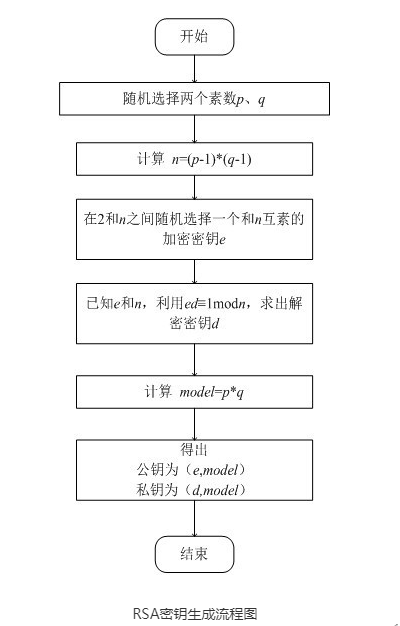（2）RSA加密部分流程图：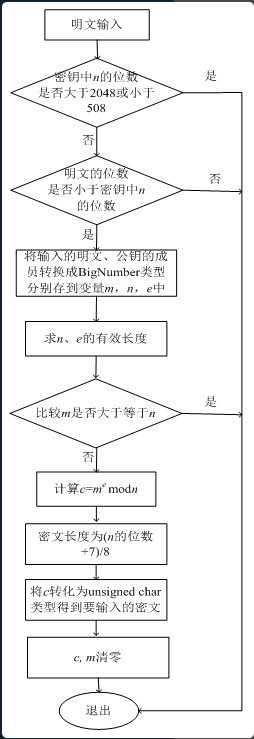（3）RSA解密部分流程图：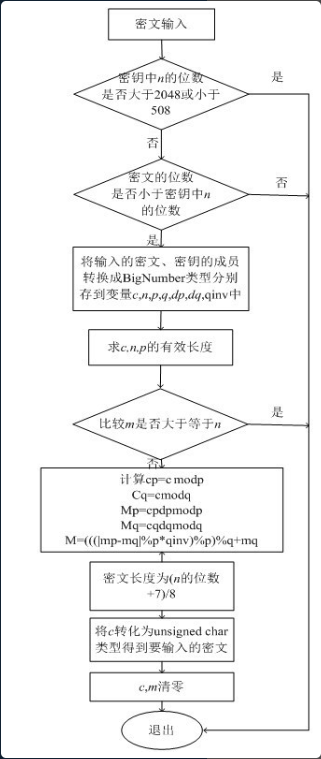源代码如下所示

大数的数据结构：

class BigNum

{

public:

int length;                      //大数长度

int signal;                      //大数符号

unsigned long array[len1];         //大数绝对值

BigNum();   //构造函数

BigNum(unsigned __int64);        //构造函数用于赋初始值

BigNum::BigNum(string s);        //读入字符串

BigNum(BigNum const& A);       //复制大数，const保护了原对象的属性

BigNum();   //析构函数

BigNum operator+(BigNum & A);    //运算符+重载

BigNum operator-(BigNum & A);    //运算符-重载

BigNum operator*(BigNum & A);    //运算符*重载

BigNum operator/(BigNum & A);    //运算符/重载

BigNum operator%(BigNum & A);   //运算符%重载

BigNum operator-(void);            //负号重载

int operator==(BigNum& A);        //等于号重载

int operator!=(BigNum& A);         //不等号重载

int Compare(BigNum const& A);     //比较两大数绝对值大小

void GeneratePrime(void);           //产生素数

int Rabin_Miller(void);              //拉宾米勒算法用于素数测试

void Random(int a);                //随机产生一个大数

void Random(BigNum A);           //随机产生一个小于A的大数

void print(void);                   //输出大数

void printS(void);                  //输出字符串

BigNum power_mod(BigNum& A, BigNum& B);   //模幂算法计算X^A mod B

BigNum ex_euclid(BigNum a,BigNum b);          //扩展欧几里德算法

RSA系统初始化部分代码：

RSA::RSA_Generated_Parameter ()｛

q.GeneratePrime();

while(p==q)                  //判断两个素数不等

q.GeneratePrime();

temp=p-I;

t=q-I;

t=t*temp;

cout<<"素数p为："<<endl;     //输出素数

p.print();

cout<<endl;

cout<<"素数q为："<<endl;

q.print();

cout<<endl;

cout<<"公钥n为："<<endl;

n=p*q;

n.print();

cout<<endl;

cout<<"公钥e为："<<endl;

e.print();

cout<<endl;

d.ex_euclid(e,t);               //计算私钥d

cout<<"私钥d为："<<endl;

d.print();

cout<<endl;

RSA加解密部分代码：

RSA::RSA_Decrypt_Crypt (char s)

{

a=BigNum(A);

cout<<endl;

b=a.power_mod(e,n);           //产生密文b

cout<<"加密结果为："<<endl;

b.print();

cout<<endl;

c=b.power_mod(d,n);           //解密

cout<<"解密后的结果为："<<endl<<endl;

c.printS();

}

【实验思考】

RSA密码算法的安全性在于什么？

RSA算法的安全在于，e、p、N，是随机生成的。

知道e和N，想寻找p，在计算上是不可行的(基于对大质数进行因式分解过于困难)。

展开全文RSA算法 编程实现
• RSA小例子，创建密钥对生成器，指定加密和解密算法RSA
• ## RSA加密算法

千次阅读 2022-04-14 15:48:30
RSA加密算法，逆元，费马小定理，扩展欧几里得，中国剩余定理

# 什么是RSA

## 一些废话

RSA是一种公钥密码算法，它的名字是由它的三位开发者，即Ron Rivest、Adi Shamir 和 Leonard Adleman 的姓氏的首字母组成的。RSA可以被用于公钥密码和数字签名。RSA是被研究得最广泛的公钥算法，从提出到现在已近三十年，经历了各种攻击的考验，逐渐为人们接受，普遍认为是目前最优秀的公钥方案之一。1983年麻省理工学院在美国为RSA算法申请了专利。

## 安全性

RSA的安全性依赖于大数分解。换句话说，RSA的难度与大数分解难度等价，一旦发现了对大整数进行质因数分解的高效算法，RSA就能够被破译。这点将在下述对 N 的讨论着重体现。

# RSA算法参数

参数解释公式描述
P、Q质数P*Q=N分解模数N后得到的值
N公共模数N=P*Q在RAS中进行模运算
E公钥指数gcd(φ,e)=11<e<φ
D私钥指数gcd(φ,d)=11<d<φ
φ欧拉公式φ=(P-1)*(Q-1)/
c密文c=me mod N/
d明文m=cd mod N/

# 参数解释

## 加密算法

由上面的参数表可知，RSA加密过程可以用下面这个公式表示

c ≡ m e mod N

也就是说，RSA的密文是对代表明文的数字 m 的 e 次方对 N 求余的结果

公钥
上述加密算法中出现的两个数—— E 和 N ，到底是什么数呢？由上面的加密算法可知，在已知明文的情况下，只要只要 E 和 N 这两个数，任何人都可以完成对加密的运算。所以说， E 和 N 是RSA加密的密钥，换句话说，E 和 N 的组合就是公钥，表示为公钥是(E,N)

公钥是可以任意公开的

## 解密算法

同样由上面的参数表可知，RSA解密过程可以用下面这个公式表示

m≡cd mod N

也就是说，RSA的明文是对代表密文的数字 c 的 d 次方对 N 求余的结果

可以发现形式上跟加密算法高度对称

私钥
参考公钥的解释，私钥也就明了了。在已知密文的情况下，只要只要 D 和 N 这两个数，任何人都可以完成对解密的运算。所以说， D 和 N 是RSA解密的密钥，换句话说，D 和 N 的组合就是私钥，表示为私钥是(D,N)

私钥必须妥善保管，不能告诉任何人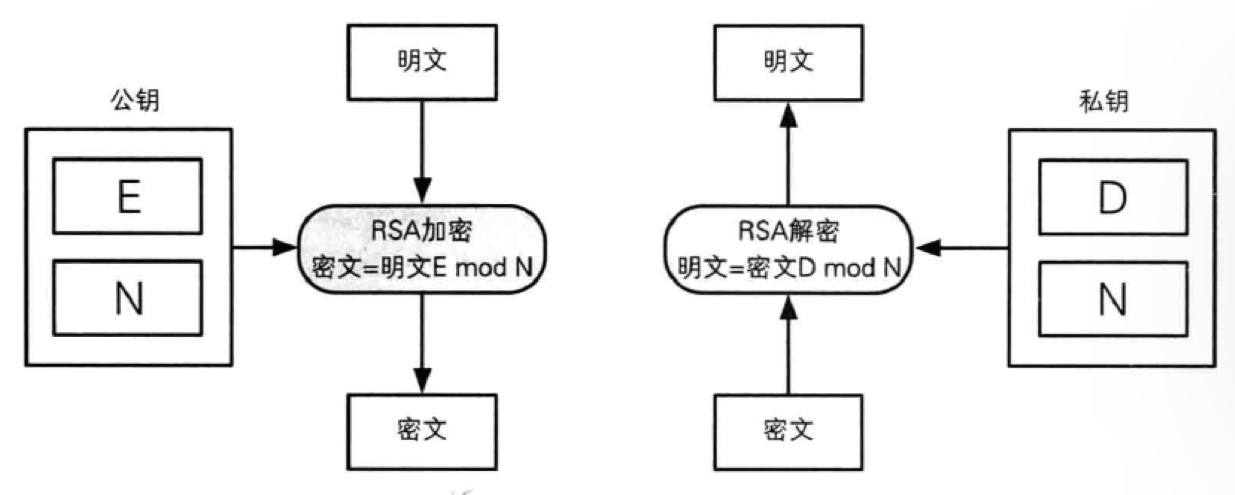## 生成密钥对

由上述分析可知，加解密的过程需要三个参数 E、D、N，那么这三个参数该怎么生成呢？由于 E 和 N 是公钥，D 和 N 是私钥，求这三个数的过程就是生成密钥对。生成步骤如下：

1、求N
2、求φ
3、求E
4、求D

求N
首先准备两个很大的质数 P、Q。上述所说的算法安全性与大数分解有关，就体现在这了，我们反过来想，如果 p 和 q 选的很小，那对于 N 的求解将会变得非常简单，密码就容易被破译；但是物极必反，也不能选的太大，太大会使得计算时间大大延长。

准备好之后，N 由以下公式求得

P*Q=N

求φ
φ这个数在加密解密的过程中都不曾出现，它只出现在生成密钥对的过程中。由下列公式求得

φ=(P-1)*(Q-1)

求E
e 的求取基于上述的 φ 值。首先明确 e 的取值范围 1<e<φ，并且 gcd(φ,e)=1（φ 与 e 的最大公约数为1，即两者互质）。之所以要加上1这个条件，是为了保证一定存在解密时需要使用的参数 D

至此，我们生成了密钥对中的公钥

求D
d 的求取基于上述的 e 值和 φ 值。首先明确 d 的取值范围 1<d<φ，并且 gcd(φ,d)=1。d 的求解方式如下

e*d mod φ=1

至此，我们生成了密钥对中的私钥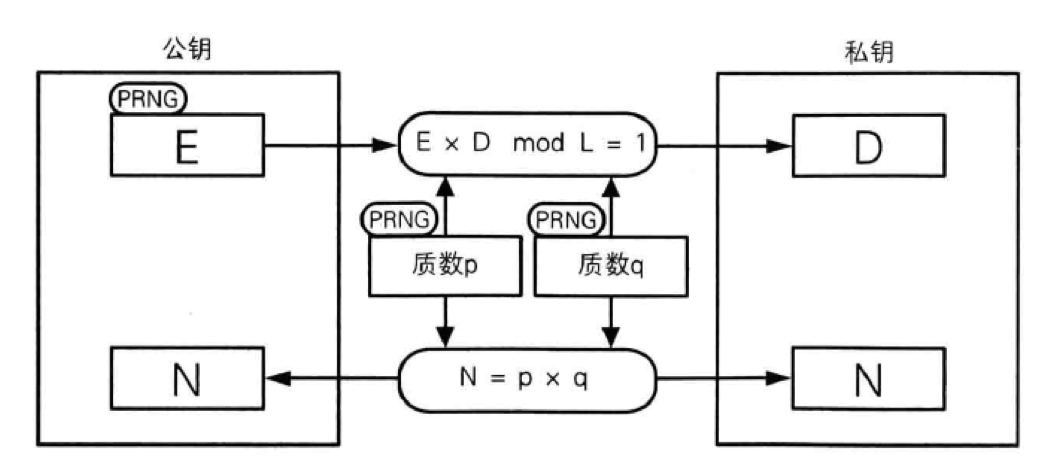## 例子

密钥对生成
① 求N
首先随便找两个素数，比如3、11(这边自己实验就取小数展示)

p = 3
q = 11

得到 N

N = 3*11 = 33

② 求φ

φ = 2*10= 20

③ 求e

gcd(φ,e)=1

满足条件的有很多，3，7，11，13，17，19… 这边我们选择3来作为 e。
至此，e = 3 ，N = 33，这就是公钥

④ 求d

e*d mod φ=1

容易得 d = 7
至此，d = 7 ，N = 33，这就是私钥

加密
要加密的明文必须是小于 N 的数，也就是小于33的数，这边就随便取一个5吧

c=me mod N = 53 mod 33 = 26

解密

m=cd mod N = 267 mod 33 = 5

## 常见大整数N的分解方法

对于常规得CTF题来说，通常会给大家公钥指数 E 和公共模数 N，而这个模数 N 是非常大得数字。我们要做的是将它分解成两个指数 p，q，进而求得 φ，再根据公式求得私钥指数，最后将密文转换成明文。

如何将大整数 N 分解呢？
1、当 N 的长度较小时，采用爆破
2、当 N 满足因数p、q相差较小或相差很大时，可以采用离线工具yafu来分解
3、当 N 的位数过大时，且不满足上述条件，可以用在线网站 factordb。该网站类似于彩虹表，将已被分解的大数结果存储起来，只需要输入查询即可。

# 逆元

逆元是什么？为什么突然讨论逆元？

还记得上面求解私钥指数d的公式吗？

e*d mod φ=1

这个公式也可以写成

e*d ≡ 1(mod φ)

## 定义

如果一个线性同余方程 ax ≡ 1(mod b)，则 x 称为 a mod b 的逆元，记作a-1 。一个数有逆元的充分必要条件是gcd(a,b)=1，此时逆元唯一存在 。

此处为什么讨论逆元呢？其一，RSA中有逆元的概念；其二，中国剩余定理(CRT)可与 RSA 算法结合来进行加解密，CRT又逃不开逆元的概念，所以就说了。逆元也是数论中一个十分重要的概念，当我们要求 (a / b) mod p的值，且a很大，大到会溢出；或者说b很大，达到会爆精度。无法直接求得a/b的值时，我们就要用到乘法逆元。

所以上述求解私钥指数d，可以说 e 的逆元是 d mod φ

## 如何求解

### 费马小定理

如果 p 是一个质数，而整数 a 不是 p 的倍数，则

a p−1 ≡ 1 (mod p)

可得

a * a p−2 ≡ 1 (mod p)

所以 a 的逆元即为 a p-2 (mod p)

之后利用快速幂求解

typedef long long ll;
ll mod = 1e9 + 7;
ll quick_pow(ll base,ll idx){
ll ans = 1;
while(idx){
if(idx & 1){
ans *= base;
ans %= mod;
}
base *= base;
base %= mod;
idx >>= 1;
}
return ans;
}

ll inv(ll a){
return quick_pow(a,mod-2);
}


### 扩展欧几里得

逆元的含义决定了ax ≡ 1(mod b)等价于ax = kb+1

void exgcd(int a, int b, int& x, int& y) {
if (b == 0) {
x = 1, y = 0;
return;
}
exgcd(b, a % b, y, x);
y -= a / b * x;
}
`

# 中国剩余定理（CRT）加速RSA算法

## CRT简介

网上介绍很多，具体可以参考中国剩余定理（孙子定理）

## 降N

前面多次讨论过了，RSA的难点就在于对于大整数 N 的分解。有没有啥方法能简化这个过程呢？

有，就是上述的中国剩余定理。由中国剩余定理可知，设 p 和 q 是互相独立的大素数，n 为 p*q，对于任意 (m1, m2)，且 0<=m1< p，0<=m2< q
必然存在一个唯一的m ，0<=m< n，使得

m1 = m mod p
m2 = m mod q

换句话说，给定一个(m1,m2)，其满足上述等式的m必定唯一存在。所以解密RSA 的流程 m = c d mod n，可以分解为

m1 = c d mod p
m2 = c d mod q

然后再计算m

但是等式 c d mod p 或者 c d mod q ，模数虽然从n降为p或q了，但是私钥指数指数d还是较大，运算还是比较消耗性能。所以我们还需要降低指数。

## 降d

仔细看等式 c d mod p

令d = k(p-1) + r 则 c d mod p
= c k(p-1)+r mod p
= c r * c k(p-1) mod p
因为 c (p-1) mod p = 1 （欧拉定理）
= c r mod p

r 是 d 除 p-1 的余数，即 r = d mod (p-1) 所以 c d mod p 可以降阶为 c d mod p-1 mod p，同理，c d mod q可以降阶为 c d mod q-1 mod q。

可令

dp = d mod p-1
dq = d mod q-1

这样 m1 和 m2 就降为

m1 = c dp mod p
m2 = c dq mod q

## 解密

模数都降的差不多了。想要求解明文，除了上述的 p、q、d、dp、dq，我们还需要 q 对 p 的逆元

qinv = q -1 mod p

结合上述的公式，我们得到最终的明文公式

h = qinv(m1−m2) mod p
m = m2+hq mod p∗q

展开全文• RSA密码算法的硬件实现，用硬件的方法实现rsa加密体制，希望对大家的学习有一顶顶的帮助。
• 大数实现的RSA密码算法，其中包含了大数的运算，素数的产生，密码算法中经常用到的有关算法,用简单的C++实现的，在VS2008平台实现的。
• RsA密码算法是目前公认的，在理论和实际应用中最为成熟和完善的一种非对称密钥体制。该文对其原理进行了介绍， 并证明了该算法的正确性。最后，使用Java语言编程实现了RSA密码算法，并进行了测试。
• pip install rsa rsaUtil.py import base64 import random import rsa # https://stuvel.eu/python-rsa-doc/usage.html#generating-keys class Key(object): def __init__(self, pubKey, priKey): self.pubKey...java git
• RSA 密码是在 TLS、SSL、IPSec 等网络安全协议中广泛使用的密码算法，其安全性至关重要。在FDTC 2014会议上，Rauzy和Guilley提出了改进的基于中国剩余定理的RSA密码实现算法，用于抵抗故障注入攻击。针对Rauzy和...RSA密码
• RSA算法，经典的非对称密码算法。该程序基本实现了RSA算法的精髓。对非对称密码学感兴趣的同学可以参考一下
• ## RSA算法详解

万次阅读 2021-01-21 11:18:30
RSA算法是目前理论和实际应用中最为成熟的和完善的公钥密码体制。RSA用来解决对称密码的密钥分发问题。还可以用来进行数字签名来保证信息的否定与抵赖，利用数字签名较容易发现攻击者对信息的非法篡改以保证信息的......